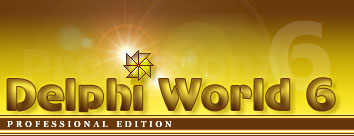﻿ Delphi World - Как найти наибольший общий делительКак найти наибольший общий делитель ```{ The greatest common factor, or GCF, is the greatest factor that divides two numbers. } uses math; // Find the greatest common factor of two integers function TForm1.GCF(A, B: Integer): Integer; var Lfactor: Integer; begin // Return -1 if either value is zero or negative if (A < 1) or (B < 1) then begin Result := -1; Exit; end; // if A = B then this is the GCF if A = B then begin Result := A; Exit; end; Result := 1; for Lfactor := trunc(max(A, B) / 2) downto 2 do begin if (frac(A / Lfactor) = 0) and (frac(B / Lfactor) = 0) then begin Result := Lfactor; Exit; // GCF has been found. No need to continue end; end; end; // Example: procedure TForm1.Button1Click(Sender: TObject); var Res: Integer; begin Res := GCF(120, 30); ShowMessage(Inttostr(Res)); end; {******************} // Find the greatest common factor of an array of integers function TForm1.GCFarray(A: array of Integer): Integer; var Llength, Lindex, Lfactor: Integer; begin Llength := Length(A); // Return -1 if any value is zero or negative for Lindex := 0 to Llength - 1 do begin if A[Lindex] < 1 then begin Result := -1; Exit; end; end; // if all elements are equal then this is the GCF Lindex := 1; while (Lindex < Llength) and (A[Lindex] = A) do Inc(Lindex); if Lindex = Llength then begin Result := A; Exit; end; Result := 1; for Lfactor := trunc(ArrayMax(A) / 2) downto 2 do begin Lindex := 0; while (Lindex < Llength) and (frac(A[Lindex] / Lfactor) = 0) do Inc(Lindex); if Lindex = Llength then begin Result := Lfactor; Exit; // GCF has been found. No need to continue end; end; end; // find the maximum value in an array of integers function TForm1.ArrayMax(Aarray: array of Integer): Integer; var Lpos: Integer; begin Result := 0; for Lpos := 0 to Length(Aarray) - 1 do if Aarray[Lpos] > Result then Result := Aarray[Lpos]; end;```
Проект Delphi World © Выпуск 2002 - 2017
Автор проекта: Эксклюзивные курсы программирования